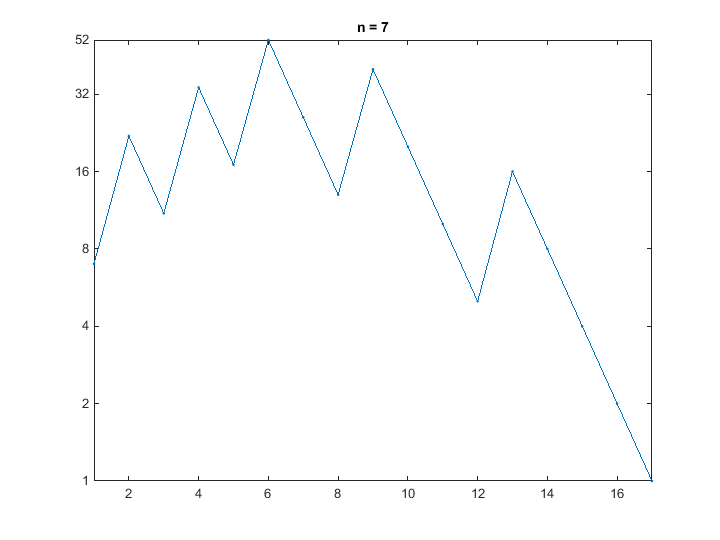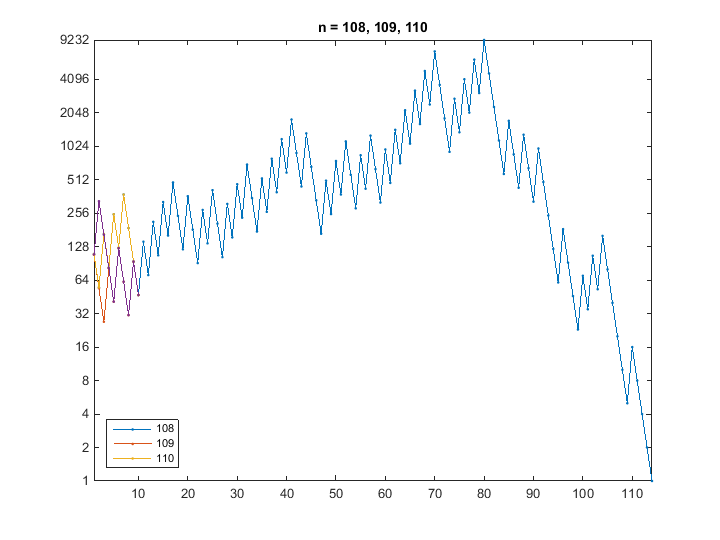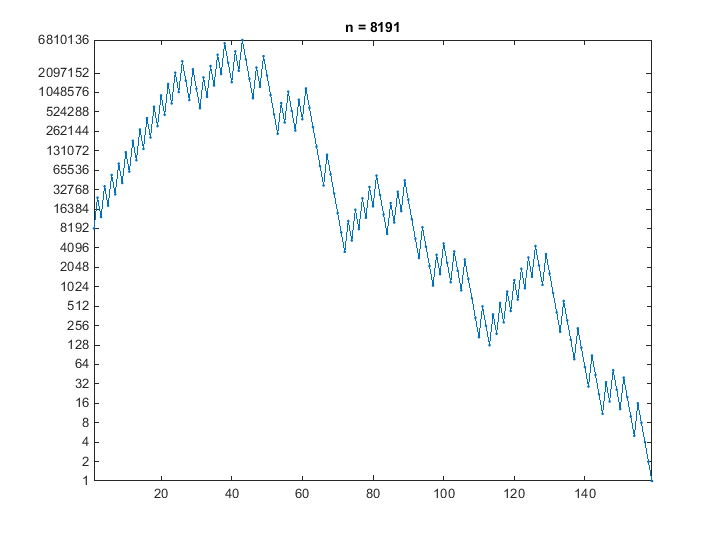# The Three n Plus One Conjecture

If $n$ is odd, replace $n$ by $3n+1$, if not, replace $n$ by $n/2$. Repeat. A famous conjecture made by Lothar Collatz is that no matter what value of $n$ is chosen to start, the process eventually terminates at $n=1$. Do not expect a proof, or a counterexample, in this blog.

### Contents

#### Function Threenplus1

You might want to download function threenplus1.m from my textbook Numerical Computing with MATLAB. This not only produces the graphs in this post, it also has a pair of uicontrols that allow you to increase and decrease the value of n. Here is the core of threenplus1. The generated sequence is collected in a vector y. We don't know ahead of time how long y is going to be. In fact, that's the point of the computation. Does the while loop terminate and, if so, how long is y?. The ability of MATLAB to grow a vector an element at a time comes in very handy here.
   dbtype 44:52 threenplus1

44    y = n;
45    while n > 1
46       if rem(n,2)==0
47          n = n/2;
48       else
49          n = 3*n+1;
50       end
51       y = [y n];
52    end


#### n = 7

A good example is provided by starting with $n = 7$. Here is the sequence collected in y. Its length is 17 and its max is 52.
   7 22 11 34 17 52 26 13 40 20 10 5 16 8 4 2 1
Here is the plot. The y-axis is logarithmic in anticipation of some fairly large values in later runs. Notice that as soon as the sequence hits a power of 2, it plunges to termination.#### n = 108, 109, 110

It is interesting to see how the graphs produced by threenplus1 change from one value of $n$ to the next. Here are the first ten elements of y for $n$ = 108, 109, and 110.
  108  54  27  82  41 124  62  31  94  47 . . .
109 328 164  82  41 124  62  31  94  47 . . .
110  55 166  83 250 125 376 188  94  47 . . .
After the first eight steps all three sequences reach the same value, so they are identical after that. The length is 114 and the max is 9232. Here are the three graphs, superimposed on one another. The similarity between the three sequences is very apparent when you use the increment n buttons provided by threenplus1.#### n = 2^13-1

Prime numbers of the form $n = 2^p-1$ where $p$ itself is prime are known as Mersenne primes. But that's a whole 'nother story. For now, suffice it to say that such numbers are candidates to produce 3n+1 sequences with a high maximum value. Here is $n = 2^{13}-1$. The sequence reaches 6,810,136 before it eventually terminates in 159 steps.I have to admit that this triggers a bug in some versions of MATLAB. If you see specious characters in the formatting of the legend on the y-axis, insert this line near the end of threenplus1.
set(gca,'yticklabel',int2str(get(gca,'ytick')'))


#### Orbits

Check out the two references below about orbits associated with the Collatz conjecture.

#### Background

When I Google "three n plus 1", the first link is to an excellent Wikipedia article on the "Collatz conjecture". According to Wikipedia, the famous German mathematican Lothar Collatz first made the conjecture, in 1937, that the process terminates for any starting value. I am already familiar with Collatz's name because of his work in numerical analysis and approximation theory, including finite difference methods for differential operators. My mentor John Todd had some contact with Collatz shortly after the end of World War II that could be the subject of a blog post some day. Many others have also been interested in the 3n+1 problem. Stanislaw Ulam (see my previous blog post), Shiszuo Kakutani, Sir Bryn Thwaites, Helmut Hasse, Paul Erdos, John Horton Conway, Douglas Hofstadter, and Richard Guy. But Wikipedia quotes Paul Erdos as saying about the Collatz conjecture: "Mathematics may not be ready for its solution."

#### References

Wikipedia, Collatz Conjecture, <http://en.wikipedia.org/wiki/Collatz_conjecture> Jason Davies, Collatz Conjecture Orbits, <http://www.jasondavies.com/collatz-graph> xkcd, Collatz Conjecture Orbits, <http://xkcd.com/710>

Published with MATLAB® R2014b
|